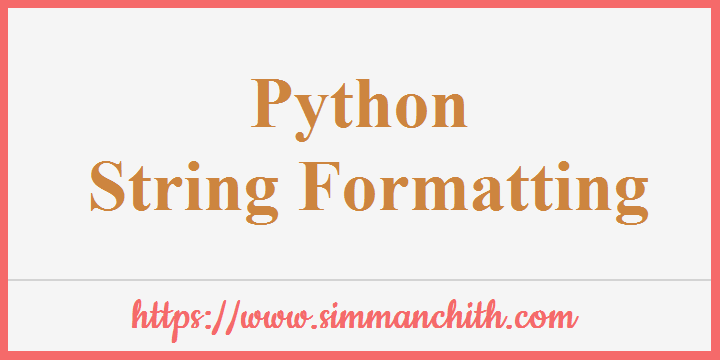# Python String FormattingWe can format the results using the `format()` method to ensure that a string is presented as expected.

## String format()

You can format chosen elements of a string using the `format()` method.

Sometimes you do not control sections of a text, perhaps it comes from a database or user input?

Add placeholders (curly brackets `{}`) in the text, and execute values via the `format()` method for controlling such values:

Example 1 :- Indicate the price by adding a placeholder :

``````price = 49
txt = "The price is {} dollars"
print(txt.format(price))``````

Output :-

The price is 49 dollars

Within the curly brackets, you can add specifications for how the value can be converted.

Example 2 :- The pricing format to be shown as a two decimal number :

``````price = 49
txt = "The price is {:.2f} dollars"
print(txt.format(price))``````

Output :-

The price is 49.00 dollars

In our `string format()` references, check all formatting types.

You can also search for these topics, python string formatting methods, operators to formatting string in python, example for python formatting the string, string ways to formatting the decimal places using python, bytes and blocks to formatting the string in python, working of python codes string formatting conditions, define the default value for string format, python String format() function.

## Multiple Values

If you wish to use more values, simply add more values to the method of `format()` :

``print(txt.format(price, itemno, count))``

Example :- Add more placeholders :

``````quantity = 3
itemno = 567
price = 49
myorder = "I want {} pieces of item number {} for {:.2f} dollars."
print(myorder.format(quantity, itemno, price))``````

Output :-

I want 3 pieces of item number 567 for 49.00 dollars.

You can also search for these topics, python multiple values return, multiple values for key in python, multiple values from python function, python multiple values in list, derive the multiple input values using python, python print and quantities with python multiple values, Example for python multiple values.

## Index Numbers

Example 1 :- You can use index numbers (a number inside the curly brackets `{0}`) to be sure the values are placed in the correct placeholders :

``````quantity = 3
itemno = 567
price = 49
myorder = "I want {0} pieces of item number {1} for {2:.2f} dollars."
print(myorder.format(quantity, itemno, price))``````

Output :-

I want 3 pieces of item number 567 for 49.00 dollars.

Example 2 :- Also, use an index number if you would like to refer to the same data more than once :

``````age = 36
name = "John"
txt = "His name is {1}. {1} is {0} years old."
print(txt.format(age, name))``````

Output :-

His name is John. John is 36 years old.

You can also search for these topics, python index numbers by condition, how to change the number of python index, count the python number of index, command the index number using python, python execute the index numbers default value, check the python error and existing of number of index, Example for python index numbers.

## Named Indexes

Example :- In the curly bracket `{carname}`, the named indexes can be used too, however when you supply the values of parameter `txt.format(carname="Ford")` you must use names :

``````myorder = "I have a {carname}, it is a {model}."
print(myorder.format(carname = "Ford", model = "Mustang"))``````

Output :-

I have a Ford, it is a Mustang.

You can also search for these topics, python indexes name change, change the name indexed event in python, named indexes using python group example, list the indexes contain names in python, how to remove named indexes with python, Example for python named indexes.